# How much heat (in kJ) is required to raise the temperature of 124.9 g of ethanol...

How much heat (in kJ) is required to raise the temperature of 124.9 g of ethanol (mw=46.07) from 30.24 °C to its boiling point of  78.37 °C and then vaporize it completely creating ethanol gas at the boiling temperature?
(specific heat = 2.46 J/g°C, ΔHvap = 48.6 kJ/mol)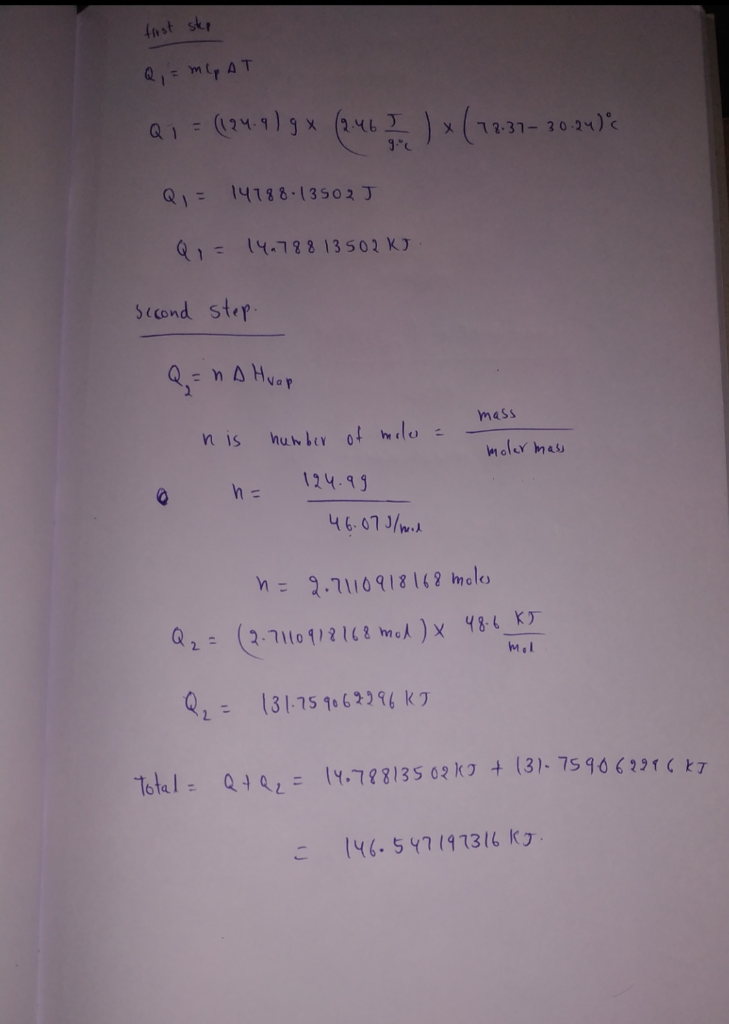#### Earn Coin

Coins can be redeemed for fabulous gifts.

Similar Homework Help Questions
• ### How much heat (in kJ) is required to raise the temperature of 202.8 g of ethanol...

How much heat (in kJ) is required to raise the temperature of 202.8 g of ethanol (mw=46.07) from 40.34 °C to its boiling point of  78.37 °C and then vaporize it completely creating ethanol gas at the boiling temperature? (specific heat = 2.46 J/g°C, ΔHvap = 48.6 kJ/mol)

• ### How much heat (in kJ) is required to raise the temperature of 288.7 g of ethanol...

How much heat (in kJ) is required to raise the temperature of 288.7 g of ethanol (mw=46.07) from 39.38 °C to its boiling point of 78.37 °C and then vaporize it completely creating ethanol gas at the boiling temperature? (specific heat = 2.46 J/g°C, ΔHvap = 48.6 kJ/mol)

• ### QUESTION 3 How much heat (in kJ) is required to raise the temperature of 122 g of ethanol (mw 46.07) from 11.29 °C to i...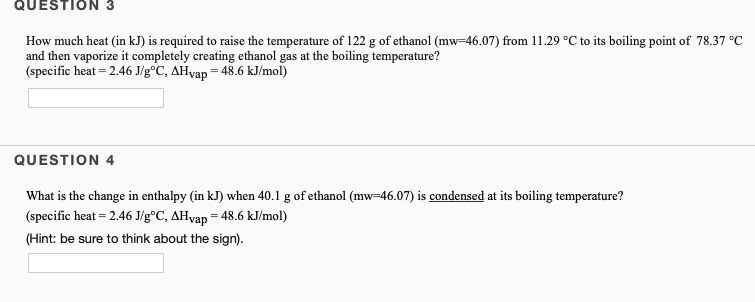QUESTION 3 How much heat (in kJ) is required to raise the temperature of 122 g of ethanol (mw 46.07) from 11.29 °C to its boiling point of 78.37 °C and then vaporize it completely creating ethanol gas at the boiling temperature? (specific heat - 2.46 J/g°C, AHyap = 48.6 kJ/mol) QUESTION 4 What is the change in enthalpy (in kJ) when 40.1 g of ethanol (mw-46.07) is condensed at its boiling temperature? (specific heat 2.46 J/g°C, AHvap 48.6 kJ/mol)...

• ### QUESTION 3 How much heat (in kJ) is required to raise the temperature of 141,4 g...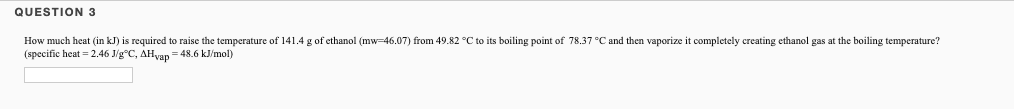QUESTION 3 How much heat (in kJ) is required to raise the temperature of 141,4 g of ethanol (mw-46,07) from 49.82 °C to its boiling point of 78.37 °C and then vaporize it completely creating ethanol gas at the boiling temperature? (specific heat=2.46 J/gºC, AH yap = 48.6 kJ/mol)

• ### a. How much heat (kJ), at 1 atm, is required to raise the temperature of 25.0...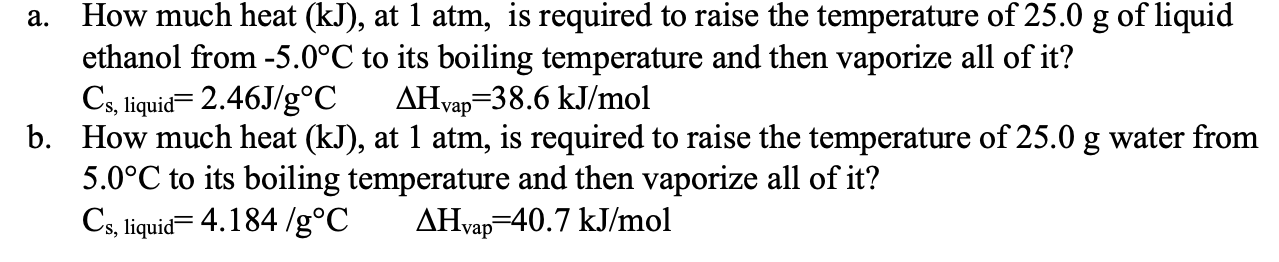a. How much heat (kJ), at 1 atm, is required to raise the temperature of 25.0 g of liquid ethanol from -5.0°C to its boiling temperature and then vaporize all of it? Cs, liquid= 2.46J/g°C AHvap=38.6 kJ/mol b. How much heat (kJ), at 1 atm, is required to raise the temperature of 25.0 g water from 5.0°C to its boiling temperature and then vaporize all of it? Cs, liquid=4.184 /g°C AHvap=40.7 kJ/mol

• ### Calculate the amount of heat (in kJ) required to raise the temperature of 14.0 g of...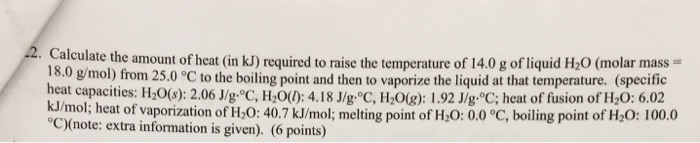Calculate the amount of heat (in kJ) required to raise the temperature of 14.0 g of liquid H_2O (molar mass = 18.0 g/mol) from 25.0 degree C to the boiling point and then to vaporize the liquid at that temperature, (specific heal capacities: H_2O(s): 2.06 J/g degree C, H_2O(l): 4.18 J/g degree C, H_2O(g): 1.92 J/g degree C; heat of fusion of H_2O: 6.02 kJ/mol; heat of vaporization of H_2O: 40.7 kJ/mol; melting point of H_2O: 0.0 degree C, boiling...

• ### Determine the amount of heat energy (in kJ) needed to warm a 58.5-g sample of ethanol...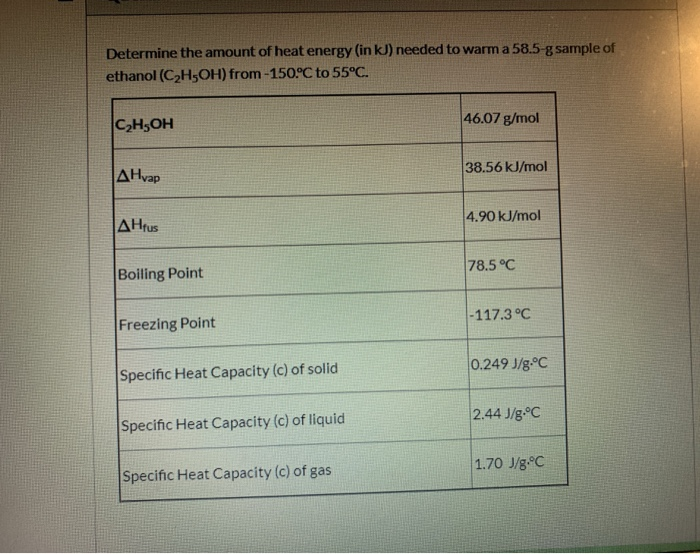Determine the amount of heat energy (in kJ) needed to warm a 58.5-g sample of ethanol (C2H5OH) from-150°C to 55°C. C2H5OH 46.07 g/mol AHvap 38.56 kJ/mol ΔΗrus 4.90 kJ/mol Boiling Point 78.5°C Freezing Point -117.3°C Specific Heat Capacity (c) of solid 0.249 J/g.°C Specific Heat Capacity (c) of liquid 2.44 J/g.°C Specific Heat Capacity (c) of gas 1.70 J/8.°C

• ### How much energy is required to raise the temperature of 33.6 g of water from -25.5°C to 86.4°C

How much energy is required to raise the temperature of 33.6 g of water from -25.5°C to 86.4°C?melting point 0.0°C boiling point 100.0°C specific heat solid 2.05 J/g · °C specfic heat liquid 4.184 J/g · °C specific heat gas 2.02 J/g · °C H° fusion 6.02 kJ/mol H° vaporization 40.7 kJ/mol

• ### How much heat energy is required to convert 15.0 g of solid ethanol at -114.5 °C...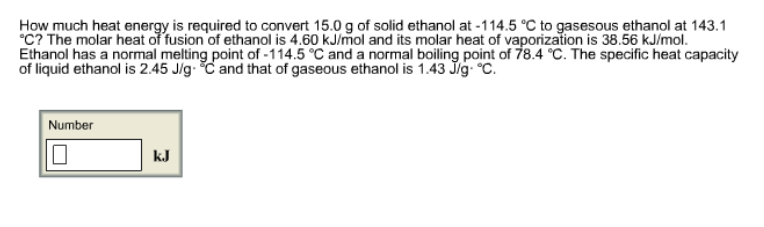How much heat energy is required to convert 15.0 g of solid ethanol at -114.5 °C to gasesous ethanol at 143.1 °C? The molar heat of fusion of ethanol is 4.60 kJ/mol and its molar heat of vaporization is 38.56 kJ/mol. Ethanol has a normal melting point of -114.5 °C and a normal boiling point of 78.4 °C. The specific heat capacity of liquid ethanol is 2.45 J/g·°C and that of gaseous ethanol is 1.43 J/g·°C. How much heat energy...

• ### What amount of thermal energy (in kJ) is required to convert 44.1 g of ethanol at...

What amount of thermal energy (in kJ) is required to convert 44.1 g of ethanol at -178 °C completely to gaseous ethanol at 119 °C? The melting point of ethanol is -114 °C and its normal boiling point is 78 °C. The heat of fusion of ethanol is 5.0 kJ mol-1 The heat of vaporization of ethanol at its normal boiling point is 35.0 kJ mol-1 The specific heat capacity of solid ethanol is 1.05 J g-1 °C-1 The specific...

Free Homework App